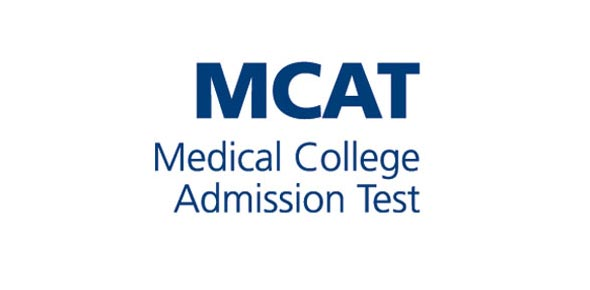# Mcqs Test For MCAT Students

30 Questions | Total Attempts: 324SettingsFor More MCAT & ECAT MCQS Knowledge

Related Topics
• 1.
Cloning is a technology for achieving
• A.

Biology aims

• B.

Scientific aims

• C.

Integrated aims

• D.

Eugenic aims

• 2.
Deductive reasoning is always from
• A.

Specific to general

• B.

Tentative to exact

• C.

General to specific

• D.

Qualitative to quantitative

• 3.
The branch of Biology dealing with social behavior and communal life of human beings is
• A.

Human Biology

• B.

Social Biology

• C.

Micro Biology

• D.

Bio technology

• 4.
The study of microorganisms is called
• A.

Parasitology

• B.

Microbiology

• C.

Social Biology

• D.

Molecular Biology

• 5.
In integrated disease management involves
• A.

Combating of disease

• B.

Loss of microbes

• C.

Extinction of species

• D.

Division of zygote

• 6.
Which one of the following is a correct sequence in biological methods
• A.

Observation-hypothesis-law-theory

• B.

Observation-hypothesis-deduction-testing of deduction

• C.

Hypothesis-observation-deduction-testing of deduction

• D.

Law-theory-observation-deduction

• 7.
A structure within a cell that perform specific function is called
• A.

Molecule

• B.

Atom

• C.

Organelle

• D.

Element

• 8.
All the living and non-living matter is formed of
• A.

Organic molecules and tissues

• B.

Atoms and sub-atomic particles

• C.

Cells and cell products

• D.

Organs and organelle

• 9.
Which of the following is not related to cloning
• A.

Replacement of the nucleus of zygote, by another nucleus of same organism

• B.

Separation of cells of embryo to form more embroys

• C.

The individuals resulting have similar genetic make up

• D.

Removal of piece of DNA or gene from the cell, and incorporating other gene or piece of DNA in it place

• 10.
AIDS is caused by
• A.

H-virus

• B.

TMV

• C.

HIV

• D.

D- virus

• 11.
An ellipse slides between two lines at right angles to one another. The locus of its centre is
• A.

A parabola

• B.

An elipse

• C.

A circle

• D.

A hyperbola

• 12.
Which of the following molecule have double covalent bond:
• A.

N2

• B.

O2

• C.

H2

• D.

Ch4

• 13.
• A.

Germanium

• B.

Aluminium

• C.

Silicon

• D.

Sodium

• 14.
As compared to pure atomic orbitals the orbitals have:
• A.

High energy

• B.

Low energy

• C.

Same energy

• D.

Variable energy

• 15.
A basic buffer solution can be prepared by mixing:
• A.

Strong acid and its salt with weak base

• B.

Weak base and its salt with strong acid

• C.

Strong base and its salt with weak acid

• D.

Weak acid and its salt with strong base

• 16.
If nP= 30 , the value of n is
• A.

6

• B.

8

• C.

10

• D.

12

• 17.
Heat of vaporization for liquids with strong dipole dipole forces will have:
• A.

Negligible values.

• B.

Reasonably high values.

• C.

Very high values.

• D.

Very low values.

• 18.
Geometrical optics was introduced by
• A.

Newton

• B.

Plato

• C.

Plato

• D.

Maxwell

• 19.
By using scientific notation 9000 can be written as
• A.

9 x 102

• B.

9 x 103

• C.

9 x 104

• D.

9 x 10-4

• 20.
X-rays are attached towards:
• A.

Cathode

• B.

Any Electrode

• C.

Anode

• D.

None of electrode

• 21.
In stoichiometric calculations
• A.

The reaction can be reversile

• B.

Side products can be formed

• C.

Law of conservation of mass may not be obeyed

• D.

Law of definite proportions is definitely obeyed

• 22.
Calcium has isotopes
• A.

7

• B.

1

• C.

8

• D.

6

• 23.
The mass of one mole of electrons is
• A.

1.008 mg

• B.

0.55 mg

• C.

0.184 mg

• D.

1.673 mg

• 24.
The number of moles of CO2which contain 8.0 g of oxygen
• A.

0.25

• B.

0.50

• C.

1.0

• D.

1.50

• 25.
The volume occupied by 1.4 g on N2at S.T.P is
• A.

2.24 dm3

• B.

22.4 dm3

• C.

112 cm3

• D.

1.12 dm3

• 26.
I molar volume of a gas at S.T.P is occupied by
• A.

1 g of gas

• B.

6 x 1023g of gas

• C.

22.4 m3of gas

• D.

1 gram molecular mass of gas

• 27.
The volume occupied by 1.6 g of O2at STP is
• A.

22.4 dm3

• B.

2.24 dm3

• C.

1.12 dm3

• D.

112 dm3

• 28.
Which of the following compound has the highest % o f oxygen by weight
• A.

CH3- OH

• B.

C2H5OH

• C.

HCOOH

• D.

H2O

• 29.
Isotopes are the atoms of same element with similar chemical properties but different
• A.

Atomic number

• B.

Atomic volume

• C.

Atomic weight

• D.

Atomic structure

• 30.
A limiting reactant is that one which
• A.

Gives greatest number of moles of products

• B.

Gives least number of moles of products

• C.

Is left behind after the completion of reaction

• D.

NOT